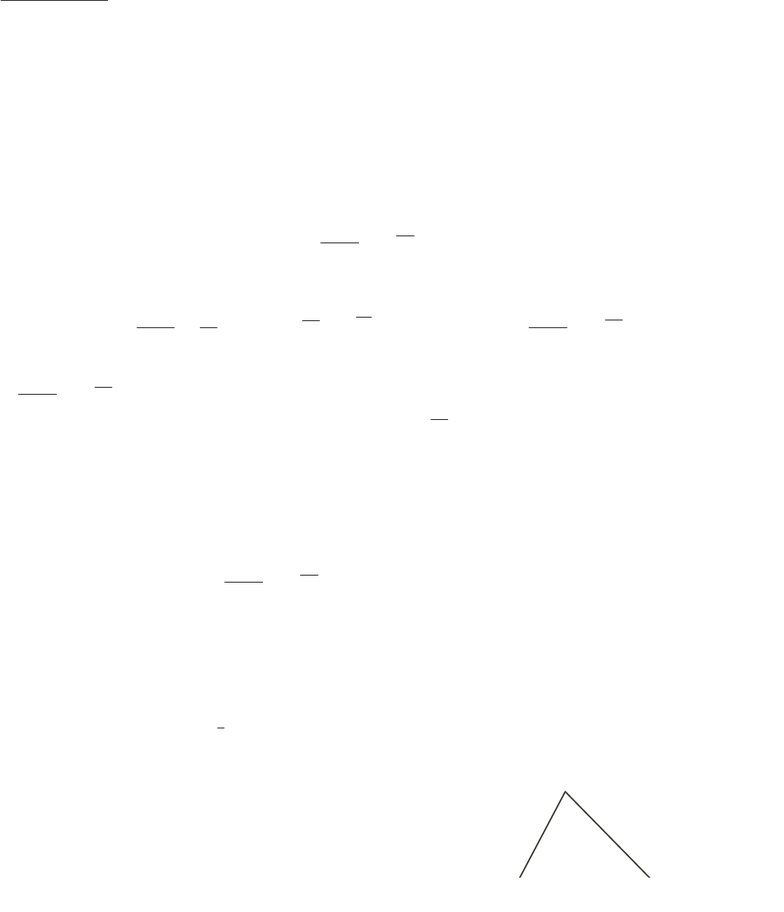Class Notes (1,100,000)
CA (650,000)
UW (20,000)
MATH (2,000)
MATH135 (300)
Lecture 9

# Lecture 9 Lecture 9, other proof styles

This preview shows half of the first page. to view the full 3 pages of the document.MATH 135 Winter 2009
Lecture IX Notes
If and only if
In mathematics, we often see statements of the form “Aif and only if B” (AB). (See Assignment
1.) This means “(If Athen B) and (If Bthen A)”. The parentheses are here for mathematical
reasons, not English language ones!
Sometimes we say “The truth of Ais equivalent to the truth of B” or “Ais equivalent to B”, since
if ABhas been proven then if Ais TRUE, Bis TRUE, and if Ais FALSE, Bcannot be TRUE
(otherwise Awould be). Can you see why?
To prove these statements, we have two directions to prove, since there are two “If...then...” state-
ments that must be proven to be TRUE.
Example
Suppose x, y 0. Then x=yif and only if x+y
2=xy.
Proof
If x=y0, then x+y
2=2x
2=xand xy =x2=x(since x0) so x+y
2=xy.
If x+y
2=xy, then
x+y= 2xy
(x+y)2= 4xy
x2+ 2xy +y2= 4xy
x22xy +y2= 0
(xy)2= 0
x=y
Therefore, x=yif and only if x+y
2=xy.
Example
In 4ABC,b=ccos(A) if and only if C= 90.
Proof
If C= 90, then cos(A) = b
c, so b=ccos(A).
Suppose b=ccos(A).
Drop a perpendicular from Bto Pon AC.
Then AP =AB cos(A) = ccos(A).
But AC =AP +P C and AC =b=ccos(A) = AP .
(Think about whether this makes sense if Pis to the right of C.)
Thus P C = 0, so Pand Ccoincide.
Therefore BCA =BP A = 90.
B
C
A
a
c
b